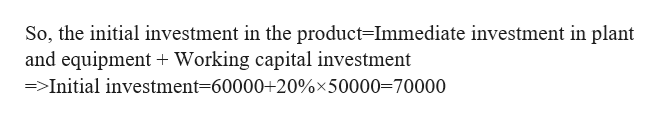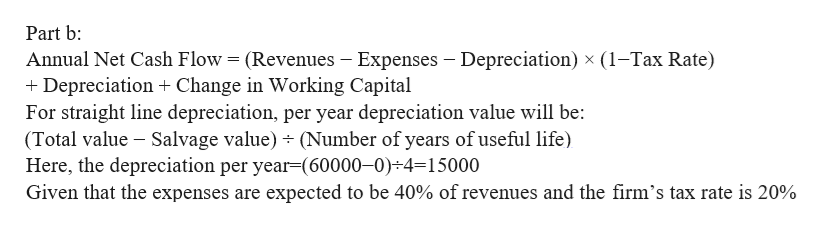# Revenues generated by a new fad product are forecast as follows:YearRevenues1\$50,000235,000330,000420,000Thereafter0Expenses are expected to be 40% of revenues, and working capital required in each year is expected to be 20% of revenues in the following year. The product requires an immediate investment of \$60,000 in plant and equipment.a. What is the initial investment in the product? Remember working capital.b. If the plant and equipment are depreciated over 4 years to a salvage value of zero using straight-line depreciation, and the firm’s tax rate is 20%, what are the project cash flows in each year? Assume the plant and equipment are worthless at the end of 4 years.c. If the opportunity cost of capital is 10%, what is the project's NPV?d. What is the project IRR?

Question
197 views

Revenues generated by a new fad product are forecast as follows:

 Year Revenues 1 \$50,000 2 35,000 3 30,000 4 20,000 Thereafter 0

Expenses are expected to be 40% of revenues, and working capital required in each year is expected to be 20% of revenues in the following year. The product requires an immediate investment of \$60,000 in plant and equipment.

a. What is the initial investment in the product? Remember working capital.

b. If the plant and equipment are depreciated over 4 years to a salvage value of zero using straight-line depreciation, and the firm’s tax rate is 20%, what are the project cash flows in each year? Assume the plant and equipment are worthless at the end of 4 years.

c. If the opportunity cost of capital is 10%, what is the project's NPV?

d. What is the project IRR?

check_circle

Step 1

Part a:
Given that the product requires an immediate investment of \$60,000 in plant and equipment.
The working capital required in each year is expected to be 20% of revenues in the following year, and the revenue in the year 1 is \$50,000.

Step 2

Answer: The initial investment in the product is \$70000help_outlineImage TranscriptioncloseSo, the initial investment in the product=Immediate investment in plant and equipment Working capital investment >Initial investment 60000+20%x50000=70000 fullscreen
Step 3help_outlineImage TranscriptionclosePart b: Annual Net Cash Flow (Revenues - Expenses Depreciation) x (1-Tax Rate) Depreciation Change in Working Capital For straight line depreciation, per year depreciation value will be: (Total value Salvage value) Number of years of useful life Here, the depreciation per year (60000-0) 4=15000 Given that the expenses are expected to be 40% of revenues and the firm's tax rate is 20% fullscreen

### Want to see the full answer?

See Solution

#### Want to see this answer and more?

Solutions are written by subject experts who are available 24/7. Questions are typically answered within 1 hour.*

See Solution
*Response times may vary by subject and question.
Tagged in